# 一、PTA实验作业（5分）

## 本周要求挑3道题目写设计思路、调试过程。设计思路用伪代码描述。题目选做要求：

### 6-3 jmu-ds- 顺序表删除重复元素

void CreateSqList(List &L,int a[],int n){//创建顺序表
L=new SqList;
L->length=-1;
int i=0;
for(i=0;i<n;i++){
L->data[i]=a[i];
L->length++;
}
}
void DispSqList(List L){//输出顺序表
int i;
for (i=0;i<=L->length;i++){
cout<<L->data[i];
if(i<L->length) cout<<" ";
}
}
void DelSameNode(List &L){//删除顺序表重复元素
int i,j,k=1,cnt=0;
for(i=0;i<=L->length;i++){
cnt=0;//cnt记录每次遍历与i相同的数
k=i+1;//
for(j=i+1;j<=L->length;j++){//遍历i以后的数
if(L->data[i]!=L->data[j]){//如果在i以后的数与i不相等
L->data[k]=L->data[j];就重建顺序表
k++;
}
else cnt++;//否则，记录相同的个数
}
L->length-=cnt; length用来记录新顺序表的长度
}
}


### 2. 设计思路（伪代码或流程图）

定义变量i,j,k.i用来遍历整个大的顺序表，j是用来嵌套遍历，k是用来新建顺序表。

for i=0 to i=length
for j=i+1 to j=length
重构顺序表，删除与i相同的数。
length=length-cnt更新每次顺序表的长度。
end for


### 2. 设计思路（伪代码或流程图）

求并集：

pa表示L1链表，p2 表示L2链表



### 2. 设计思路（伪代码或流程图）

定义一个n表示输入的公共长度，定义结构体指针，L1，L2，L，L用来表示合并后的新链表
<1>创建链表L1，L2。过程省略不详。
<2>合并链表 ：定义结构体指针p1,p2,r,s。p1=L1,p2=L2

<3>寻找中位数：![](https://images2018.cnblogs.com/blog/1233828/201803/1233828-20180325221446243-267661662.png)

让n=j/2;
for i=0 to n
p=p->next;



## 3.代码截图（注意，截图，截图，截图。不要粘贴博客上。不用用···语法去渲染）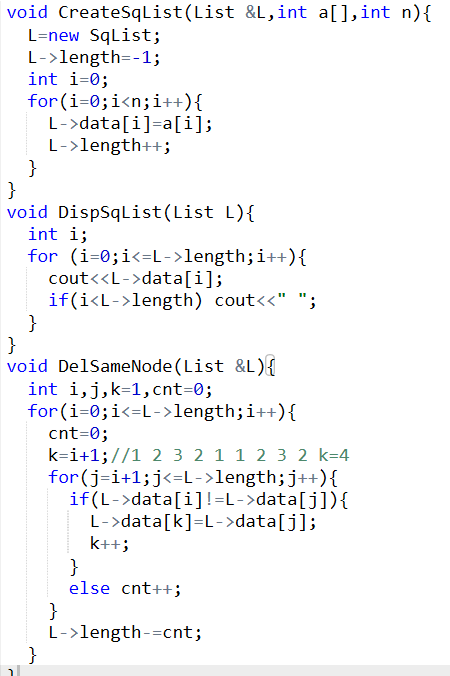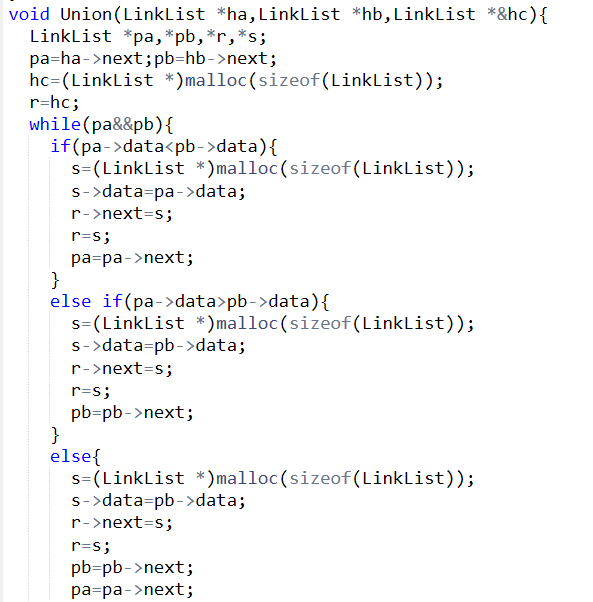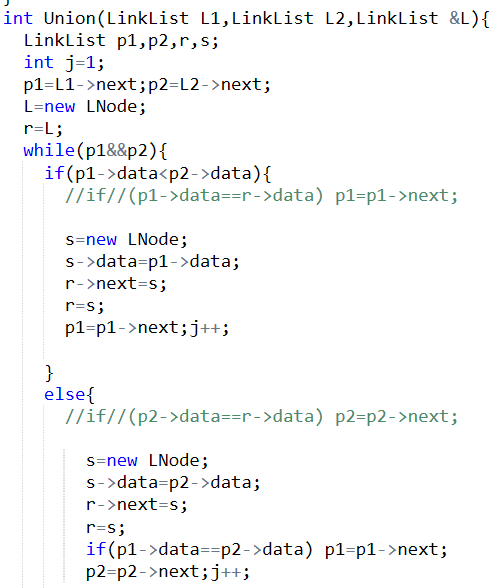## 4.PTA提交列表说明。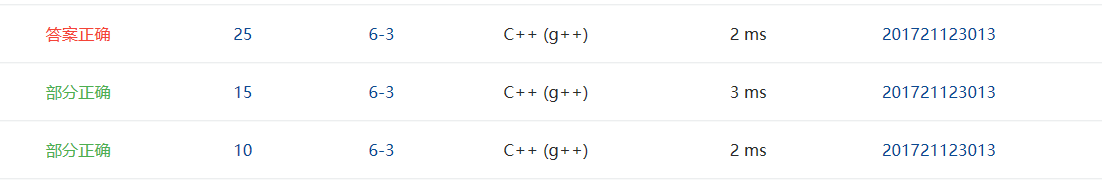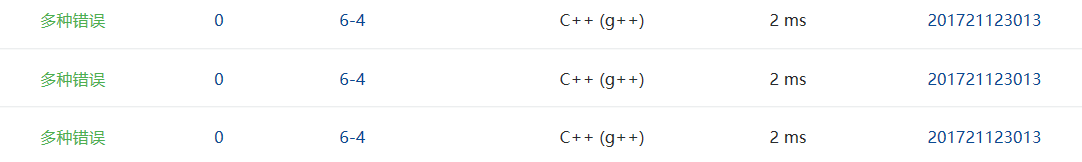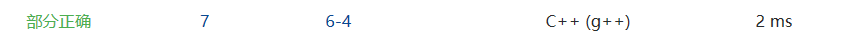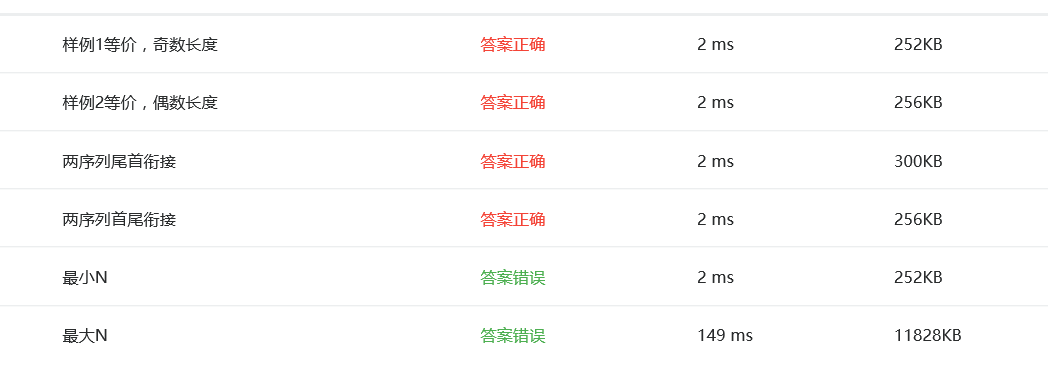# 二、截图本周题目集的PTA最后排名（3分）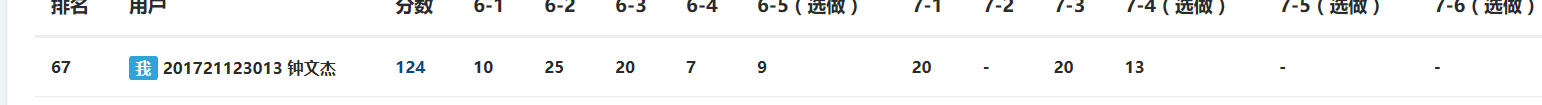# 三、本周学习总结（2分）

## 3.代码Git提交记录截图

posted @ 2018-03-25 22:50  z&jack  阅读(286)  评论(2编辑  收藏  举报